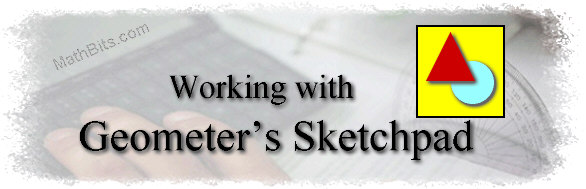Basic "How-To" Directions for Using GSP:
(Beginning directions for students who are starting to learn to make their own GSP drawings.)
(Directions for use with Geometer's Sketchpad 4.0 or higher.)

 1. Set Preferences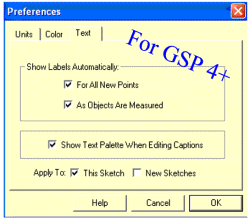For GSP 4.+ - Choose EDIT, Preferences.  Under the Units tab, set the angle measure to degrees.  Under the Text tab, under "Show Labels Automatically", check the box "For All New Points" to engage the automatic labeling of points.  (If you would rather label the points on your own, do not engage automatic labeling.  Simply use the Text tool (the "A") to label points as needed.) 2. Construct a Line Segment: a.  Using the point tool,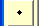, hold down the shift key and plot 2 points.  Holding the shift key down will insure that the points remain "selected" for the next step.  Clicking sequentially will also select objects. b.  Under the CONSTRUCT menu, choose SEGMENT.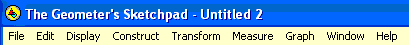3. Construct a Polygon: a.  Using the point tool, holding down the shift key and plot points in either a clockwise or counter-clockwise direction. b.  Select SEGMENT under the CONSTRUCT menu and a polygon will be drawn. 4. Construct a Diagonal: a.  Using the select tool,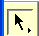, select (by clicking) 2 non-consecutive vertices. b.  Under the CONSTRUCT menu, choose SEGMENT to form a diagonal. 5. Use the Calculator: a.  Under the MEASURE menu select CALCULATE. b.  Reminder:  The asterisk (*) is used for multiplication. 6. Calculate the Measure of an Angle: a.  Using the select tool, select three consecutive vertices of the polygon.  (Remember the vertex should be selected as the second point.) b.  Under the MEASURE menu, select ANGLE. 7. Calculate the Sum of the Angles: a.  Select CALCULATE from the MEASURE menu. b.  Select each measurement on the sketch.  As a measurement is selected, it will appear in the calculator.  Enter the "plus" between measurements to create the sum. c.  Hit OK and the sum will appear in your sketch.